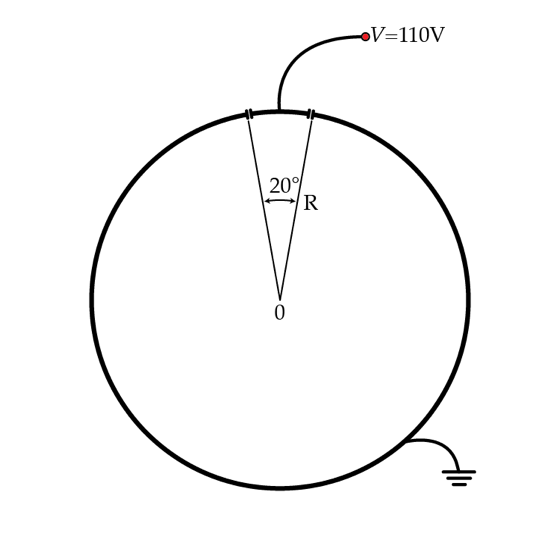# Jackson Style

Consider a very long hollow metallic cylinder of radius $R$. A section of the cylinder (corresponding to $\theta=20^{\circ}$) is kept at constant potential $V=110~\mbox{V}$ while the rest of the cylinder is kept at $0~\mbox{V}$ (grounded) as shown in the figure. Find the potential $V_{axis}$ in Volts on the axis of the cylinder (point O).×

Problem Loading...

Note Loading...

Set Loading...